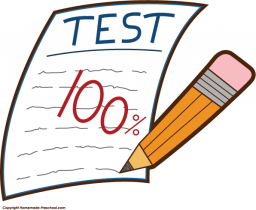# Pass a test

The student has to pass a test that contains ten questions. For each of them, he chooses one of 5 answers, with just one being correct. The student did not prepare for the test, so he randomly chose the answers. What are the probabilities that the student will answer correctly:
a) a maximum of 3 questions
b) at least 4 questions
c) all ten questions

a =  0.8791
b =  0.1209
c =  0

### Step-by-step explanation:Did you find an error or inaccuracy? Feel free to write us. Thank you!

Tips for related online calculators
Looking for a statistical calculator?
Would you like to compute the count of combinations?